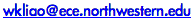# Parallel K-Means Data Clustering

This software package parallel-kmeans.tar.gz (4.6 MB) of parallel K-means data clustering contains the followings:
• A parallel implementation using OpenMP and C
• A parallel implementation using MPI and C
• A sequential version in C
For large data support (more than 2 billion number of data points), see this page for an MPI implementation that uses 8-byte integers.

### Algorithm:• The above sequential algorithm is based on the paper by MacQueen, J. "Some Methods for Classification and Analysis of Multivariate Observations." In the 5th Berkeley Symposium on Mathematical Statistics and Probability, 1967.
• The parallel implementation uses data parallelism. Data objects to be clustered are evenly partitioned among all processes while the cluster centers are replicated. Global-sum reduction for all cluster centers is performed at the end of each iteration in order to generate the new cluster centers.

### To compile:

Although I used Intel C compiler, icc, version 7.1 during the code development, there is no particular features required except for OpenMP. Thus, the implementation should be fairly portable. Please modify Makefile to change the compiler if needed.

• Make command for the sequential version: make seq_main
• Make command for the MPI version: make mpi_main
• Make command for the OpenMP version: make omp_main
• To enable the feature of using parallel netCDF to performance I/O, edit Makefile to change variable ENABLE_PNETCDF to yes/no and set the variable PNETCDF_DIR to the place where parallel netCDF is installed.

### To run:

• The Makefile will produce executables
• "omp_main" for OpenMP version
• "mpi_main" for MPI version
• "seq_main" for sequential version
• The list of available command-line arguments can be obtained by running -h option
• For example, running command "omp_main -h" will produce:
```Usage: main [switches] -i filename -n num_clusters
-i filename    : file containing data to be clustered
-c centers     : file containing initial centers. default: filename
-b             : input file is in binary format (default no)
-r             : output file in binary format (default no)
-n num_clusters: number of clusters (K must > 1)
-t threshold   : threshold value (default 0.0010)
-p nproc       : number of threads (default system allocated)
-a             : perform atomic OpenMP pragma (default no)
-o             : output timing results (default no)
-v var_name    : using PnetCDF for file input and output and var_name
is variable name in the netCDF file to be clustered
-k var_name    : name of variable in the netCDF to be used as the
initial cluster centers. If skipped, the variable
name from the option "-v" is used
-d             : enable debug mode
-h             : print this help information
```

### Input file format:

The executables read an input file that stores the data points to be clustered. A few example files are provided in the sub-directory ./Image_data. The input files can be in two formats: ASCII text and raw binary.

• ASCII text format:
• Each line contains the ID and coordinates of a single data point
• The number of coordinates must be equal for all data points
• Example files: color100.txt, texture100.txt, and edge100.txt
• Raw binary format:
• File header contains two 4-byte integers.
• The first 4-byte integer is the number of data points.
• The second integer is the number of coordinates for each data point.
• The rest of the file (file body) contains the coordinates of all data points and each coordinate is a 4-byte floating point of type float.
• There are three example files (in little Endian format) provided: color17695.bin, edge17695.bin, and texture17695.bin. They each contains 17695 data points.
• NetCDF file format:
• Note: The first K elements of the input data are picked as the initial K cluster center coordinates.

### Output files:

There are two output files:

• Coordinates of cluster centers
• The file name is the input file name appended with ".cluster_centres".
• The default is in ASCII text format.
• Each line contains an integer indicating the cluster id and the coordinates of the cluster center.
• Binary format can be enabled by using -r option.
• Header: the first 4-byte integer is the number of clusters and the second 4-byte integer is the number of coordinates of the cluster center.
• Body: the coordinates of all cluster centers.
• Membership of all data points to the clusters
• The file name is the input file name appended with ".membership".
• The default is in ASCII text format.
• Each line contains two integers: data point index (from 0 to the number of points) and the cluster id indicating the membership of the point.
• Binary format can be enabled by using -r option.
• Header: a 4-byte integer for the number of data points.
• Body: the list of cluster IDs (4-byte integers), corresponding to each data point.

### Performance results:• Performance results on Hopper, the Cray XE6 at NERSC, 2011. Hopper has 153,216 processors cores (6,384 compute nodes made up of 2 twelve-core AMD MagnyCours 2.1 GHz processors). The input data contains 1 billion data points and each data point is a vector of 128 dimensions.

### Limitations:

• Data type -- This implementation uses C float data type for all coordinates and other real numbers.
• Large number of data points -- The number of data points cannot exceed 2G due to the 4-byte integers used in the programs.

### Derived Work:

Please send comments to